Midterm 1 Preparation

# Midterm 1: Version B

1. Evaluate: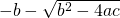if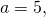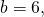and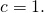2. Solve for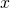in the equation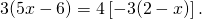3. Isolate the variable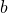in the equation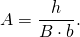4. Solve forin the equation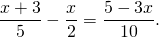5. Find the equation of the horizontal line that passes through the point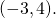6. Find the equation that has a slope of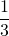and passes through the point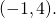7. Find the equation of the line passing through the points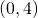and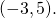8. Graph the relation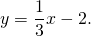For questions 9 to 11, find each solution set and graph it.

1.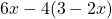>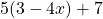2.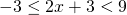3.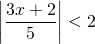4. Graph the relation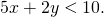5. Find two consecutive even integers such that their sum is 16 less than five times the first integer.
6. Karl is going to cut a 40 cm cable into 2 pieces. If the first piece is to be 4 times as long as the second piece, find the length of each piece.
7.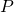varies directly as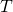and inversely as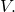If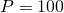when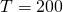and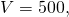find the constant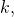then use this to findwhen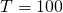and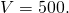<a class=”internal” href=”/intermediatealgebraberg/back-matter/midterm-one-version-b-answer-key/”>Midterm 1: Version B Answer Key